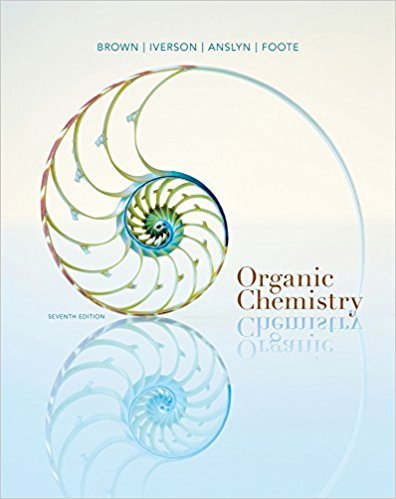×
Get Full Access to Organic Chemistry - 7 Edition - Chapter 18 - Problem 18.69
Get Full Access to Organic Chemistry - 7 Edition - Chapter 18 - Problem 18.69

×

# Chloroformates have the functional groupOR O Cl, in which R is often a tert-butylorISBN: 9781133952848 483

## Solution for problem 18.69 Chapter 18

Organic Chemistry | 7th Edition

• Textbook Solutions
• 2901 Step-by-step solutions solved by professors and subject experts
• Get 24/7 help from StudySoup virtual teaching assistantsOrganic Chemistry | 7th Edition

4 5 1 377 Reviews
31
0
Problem 18.69

Chloroformates have the functional groupOR O Cl, in which R is often a tert-butylor benzyl group. A chloroformate is used in the following synthesis of the antibacterialdrug linezolid (Zyvox).

Step-by-Step Solution:
Step 1 of 3

I. Chapter 11 Continued A. Concentration Units 1. Molarity (M) a. M = moles/liters b. [KCl] means molarity of a KCl solution 2. Molality (m) a. m = moles/kg of solvent b. mass-based and independent of temperature 3. Mass Fraction a. Mass fraction = mass of solute/total mass b. Mass percent = mass fraction * 100% 4. Mass Percent a. Mass percent is parts per hundred i. Ppm = part per million ii. Ppb = parts per billion iii. Ppt = parts per trillion b. 1 ppm of solute in water = 1 mg/1000g of solution i. Since 1 L of H2O has a mass of 1000 g: 1. 1 ppm = 1 mg/L 2. 1 ppb = 1 ug/L 3. 1 ppt = 1 ng/L 5. Problems: B. Colligative Properties 1. Dependent on number of solute “units” in solution 2. Particle type is unimportant a. Ex. Vapor pressure -> Solvent of vapor pressure is lowered by dissolved solvents C. Raolt’s Law 1. A nonvolatile solute in a volatile solvent obeys: a. Psolution = xsolvent * Psolvent b. Xsubi is mole fraction c. Lower purity solvent = lower vapor pressure 2. Problems: D. Van’t Hoff Factor 1. Ionic compounds break apart in water

Step 2 of 3

Step 3 of 3

##### ISBN: 9781133952848

The full step-by-step solution to problem: 18.69 from chapter: 18 was answered by , our top Chemistry solution expert on 03/16/18, 05:02PM. This full solution covers the following key subjects: . This expansive textbook survival guide covers 24 chapters, and 1131 solutions. Organic Chemistry was written by and is associated to the ISBN: 9781133952848. This textbook survival guide was created for the textbook: Organic Chemistry, edition: 7. The answer to “Chloroformates have the functional groupOR O Cl, in which R is often a tert-butylor benzyl group. A chloroformate is used in the following synthesis of the antibacterialdrug linezolid (Zyvox).” is broken down into a number of easy to follow steps, and 29 words. Since the solution to 18.69 from 18 chapter was answered, more than 246 students have viewed the full step-by-step answer.

## Discover and learn what students are asking

Statistics: Informed Decisions Using Data : Inference about the Difference between Two Medians: Dependent Samples
?In Problems 3–10, use the Wilcoxon matched-pairs signedranks test to test the given hypotheses at the a = 0.05 level of significance. The dependent sa

Unlock Textbook Solution